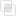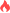python多进程如何优化显示进度条

Python 多进程2021-09-13 10:05:05687浏览 · 0收藏 · 0评论1、利用multiprocessing进程池的imap方法，将函数依次作用于可迭代对象的所有元素，并发送到多个进程。

2、配合tqdm库，可以通过进度条显示多进程代码的整体执行进度。

```from multiprocessing import Pool
from tqdm import tqdm
import math
import numpy as np

def func(x):
return math.sin(x)+math.cos(x)
with Pool(processes = 20) as pool:
result = list(tqdm(pool.imap(func, np.linspace(0,2*math.pi,1000)), total=1000))```python requests读取服务器响应python requests响应内容的三种方法python requests发送不同类型的数据python requests检测响应状态码python requests重定向的操作python requests的超时使用python捕获异常的原因python创建平衡二叉树的方法python如何配置文件路径python字典的底层原理python函数中的形参有几种python函数实参的四种类型python变量名的查找方法python global的创建和修改python格式化经纬度的方法css中transform函数的使用290

css选择器之间的关系132

css超链接是什么307